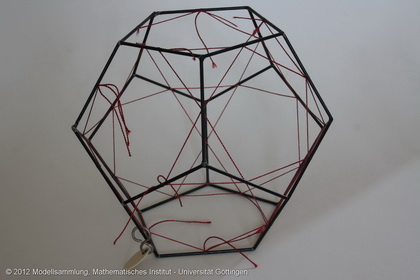Göttingen Collection of Mathematical Models and Instruments

Dodecahedron with inscribed icosidodecahedron

Model 480Category: B II 185

Description

Dodecahedron (black wire) with inscribed (12+20)-plane polygon with 30 vertices (red fiber), polar figure of the rhombus triacontrahedron.

Dodecahedron  The dodecahedron is one of the five Platonic solids, see also model 702.

Inscribed (12+20)-plane polygon with 30 vertices The solid inscribed in the dodecahedron is a icosidodecahedron. The icosidodecahedron arises from the dodecahedron by truncating its vertices. By truncating the vertices to the middle of the edges 20 new triangles and the previously existing 12 hexagons are transformed to 12 smaller hexagons. The icosidodecahedron is circumscribed by

20 triangles + 12 pentagons = 32 faces.

It has 30 vertices and 60 edges. At each vertice two triangles and two pentagons are meeting (3,3,5,5).

The icosidodecahedron is one of 13 Archimedean solids, see also 482.

rhombic triacontahedron The icosidodecahedron is polar (dual) to the rhombic triacontahedron, see also model 926. To create this new solid a sphere is inscribed in the icosidodecahedron such that the sphere touches each of the faces in exactly one point. These points of contact create the vertices of the dual solid. By connecting these 32 vertices 30 rhombi are formed. These rhombi are the faces of the rhombic triacontahedron. The number of edges remains the same during the transformation into the dual solid, while the number of vertices and faces is exchanged.

There are 11 Archimedean solids in the collection.

 472 Truncated tetrahedron inscribed in a tetrahedron 473 Truncated octahedron inscribed in an octahedron 474485 Cuboctahedron 474 inscribed in an octahedron485 inscribed in a cube 475 Truncated cube inscribed in an octahedron 476 Rhombicuboctahedron inscribed in a cube 478 Truncated icosahedron inscribed in an icosahedron 479 truncated dodecahedron inscribed in a dodecahedron 480481 Icosidodecahedron 480 inscribed in a dodecahedron481 inscribed in an icosahedron 482 Rhombicosidodecahedron 483 Snub dodecahedron 484 Truncated cube inscribed in a cube

The truncated icosidodecahedron and the lost truncated cuboctahedron are not included in the collection.

Showcase of this model is Case number U 7

References

Heesch, H.. Comm.Math.Helv, 6, n=4, Case 3a_2, fig.13.

Hess, E.(1883). Kugelteilung, Teubner, mit Figuren, §33, fig. 19,29,30.